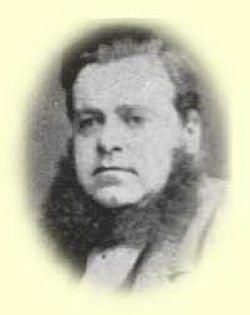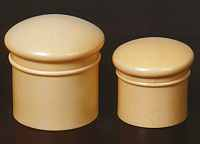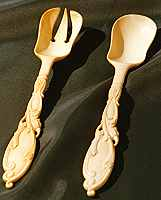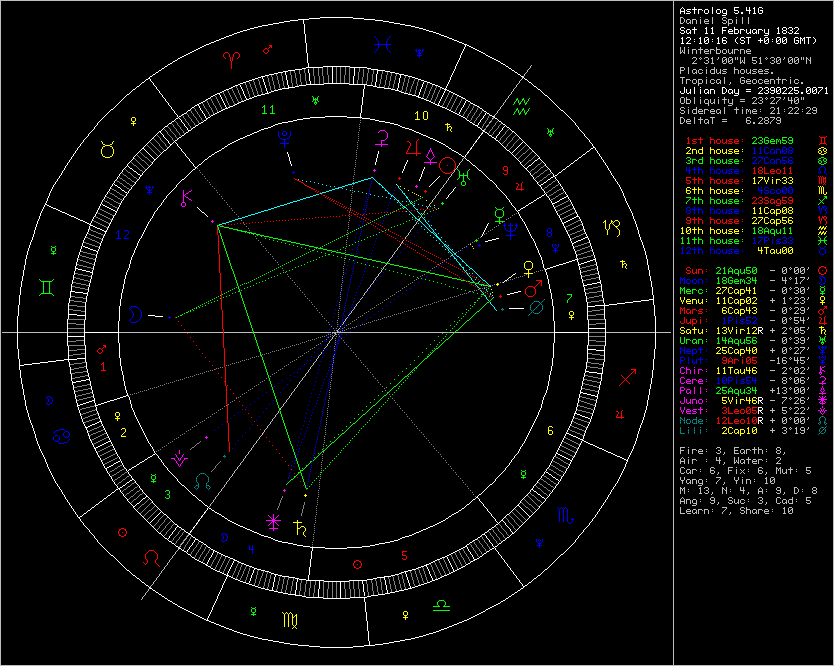Daniel SpillHaumea/Eris/Sun conjunction

>>Daniel Spill (11 February 18321887) was born in Winterbourne in Gloucestershire, England. He married at the age of 18; his bride was 15.
Although he trained as a doctor he joined the business of his brother George. The firm of George Spill & Co. manufactured waterproof textiles in Stepney Green, East London by spreading rubber onto cloth. The material was much in demand for capes and groundsheets for soldiers in the wet conditions of the Crimean War.
Spill became aware of Parkes' claim for the waterproof qualities of Parkesine probably at the 1862 exhibition. Negotiations led to an agreement not only to use it for waterproofing but also to develop Parkesine in the works of George Spill at Hackney Wick.
A provisional patent was granted in 1863 to the Spill brothers and Thomas James Briggs concerning "improvements in the manufacture of driving straps or bands and of flexible tubes or hose".
In 1866, the Parkesine Co. was established with Daniel Spill as works manager and Parkes as managing director. The Company did not prosper and was wound up in 1868, Spill taking over most of the stock. He formed the Xylonite Co. in 1869 to carry on the business but that did not fare much better and was wound up in 1874.
Undaunted by these failures, Spill established Daniel Spill & Co. in Homerton, continuing to make Xylonite and Ivoride. This enterprise succeeded in that others entered into an agreement with Spill in 1877 to form the British Xylonite Co. in the same premises. This company did go on and prosper.Some objects made with Xylonite

Spill's later years were largely occupied in a long legal battle in America with Hyatt and the Celluloid Corp. for infringement of his patents. The lawsuit was filed in 1875, found in his favour in 1880, but reversed in 1884. Spill returned to England and in 1887 died of diabetes at the age of 55.
For more than 20 years Spill had doggedly pursued the goal of making a successful business from Parkes' invention.
In 1881 Spill was listed as a retired manufacturer in the 1881 census of Leyton High Street, living with his wife and daughter and his nephew George,an India Rubber Manufacturer.<<

http://en.wikipedia.org/wiki/Daniel_Spill

_________________________________________________

Assuming noon in Winterbourne, Gloucestershire, England (51° 29' 53 N, 2° 31' W), converted to 12:10:16 UT by Astrodienst.

Using RIYAL 3.1

Astrological Setting (Tropical - Placidus)RIYAL  Sat February 11 1832  UT 12h10m16s  Lat51n30  Lon2w31   SORT ALL

Planet        Longit.      Latit.    Declin.  Const.

UJ438    =  0Aq06      2n12   17s59   Cap
BU48     =  0Li36 r   11n07    9n57      Vir
KF77     =  1Le03 r    4n13   24n03   Cnc
Ixion    =  1Ta09     11s39    0n56      Cet
VQ94     =  1Ar11     55s19   48s34   Eri
RN43     =  1Ca28 r   19s34    3n53   Ori
WN188    =  1Ar31     23s07   20s31   Cet
CF119    =  1Ar43     17n16   16n29   Peg
Jupiter  =  1Pi52      0s54   11s40     Aqr
AZ84     =  2Sc04 r    8s44   20s23    Vir
PA44     =  2Le12 r    3s26   16n20    Cnc
CY118    =  2Ta19     20s39    7s08    Cet
UR163    =  2Vi20 r    0s25   10n16    Leo
CR105    =  2Pi23     10s31   20s25   Aqr
OO67     =  2Sc29 r   19n12    5n44   Vir
OM67     =  2Li38 r   14s50   14s37   Crt
Pelion   =  3Ar21      7s49      5s50     Cet
Logos    =  3Aq49      0n21   18s58    Cap
Amycus   =  3Ta52     13n06    25n05   Tri
XZ255    =  4Sc03 r    1n54   11s06     Vir
SB60     =  4Ca09 r    1n11   24n35     Gem
RL43     =  4Sc41 r    9n51    3s48      Vir
96PW     =  4Vi43 r    6n57   16n15     Leo
FZ53     =  4Pi44     25n29   13n58     Peg
Cyllarus =  4Sa51      2s50   23s54     Sco
TC302    =  5Cp08     33s31   56s50   Tel
GV9      =   5Pi45     2 1n31    10n38    Peg
TO66     =  6Le16 r   20n58   38n55     Lyn
Crantor  =  6Sc23     12n57    1s24     Vir
Elatus   =  6Vi42 r    5n29     14n09    Leo
Mars     =  6Cp43      0s29   23s46    Sgr
RP120    =  7Sc01 r    0s24   14s14   Lib
CO104    =  7Pi55      0s39    9s13    Aqr
MW12     =  8Ge07 r   20s12    1n43   Ori
Ceto     =    8Pi14       4n33     4s16    Aqr
RD215    =  8Sc35 r    4n54    9s43   Lib
RG33     =  8Ca43 r   36n57   59n59   Lyn
Deucalion=  9Ar03      0s14    3n22   Psc
Quaoar   =  9Ta04      4s28   10n18   Ari
Pluto   =  9Ar05     16s45   11s47     Cet
GM137    =  9Ta31     11s31    3n44   Cet
TY364    =  9Le43 r    3s52   14n07   Cnc
Vertex   = 10Sc42
PJ30     = 10Ca43 r    1n01   24n03   Gem
Okyrhoe  = 10Ar45      5s34    0s52   Cet
OX3      = 10Pi55      3n02    4s40      Aqr
PB112    = 10Sc58 r   11s14   25s46   Hya
Venus    = 11Cp02      1n23   21s37   Sgr
KX14     = 11Pi11      0n20    7s04     Aqr
Echeclus = 11Li34 r    1n10    3s30   Vir
XA255    = 11Ge42 r    6s38   15n38   Tau
Chiron   = 11Ta46      2s02   13n26    Ari
QF6      = 12Aq10      5n08   12s14   Aqr
QB1      = 12Vi12 r    0n35    7n32      Leo
Node     = 12Le28      0n00   17n05   Cnc
VR130    = 12Sc54 r    2n22   13s28   Lib
Saturn   = 13Vi12 r    2n05    8n32    Leo
Orcus    = 13Sc40     14s38   29s51   Cen
UX25     = 13Li57 r    3s22     8s36     Vir
Nessus   = 14Ta00      4n33   20n24   Ari
Uranus   = 14Aq56      0s39   16s59   Cap
DH5      =  14Cp57     17n23    5s20    Aql
PN34     = 15Aq23      4n56   11s31    Aqr
TD10     = 15Li34 r     1n18     4s56    Vir
RZ214    = 15Li48 r    6s13   11s57    Vir
Thereus  = 16Ge13 r   17s29    5n20   Ori
Hylonome = 16Ta54      3s19   13n43   Ari
TL66       = 16Sa56      16n13    6s40   Oph
Teharonhi= 17Ca04 r    0n39   23n00   Gem
HB57     = 17Ge36 r   13s53    9n03    Ori
(Midheav)  = 18Aq11      0n00   15s24   Cap
TX300    = 18Le17 r    1n55   17n11    Leo
DA62     = 18Ca31 r   60n19   79n42   Dra
(Moon)     = 18Ge34      4s17   18n42   Tau
WL7      = 19Ge35 r   11n22   34n23   Aur
VS2      = 19Vi53 r    11s29    6s33     Crt
Pholus   = 20Sa17     15n50    7s18   Oph
OP32     = 21Ge05 r   27s12    3s59   Ori
XR190    = 21Cp22     34n13   12n05   Aql
FP185    = 21Ta25     26s01    7s03   Eri
CE10     = 21Li25 r   30s15   36s00   Cen
RM43    = 21Sa48      0n33   22s40   Oph
Sun      = 21Aq50      0n00   14s14     Cap
Chariklo = 21Cp53      3s21   24s59   Sgr
Typhon   = 21Cp56      2s01   23s39   Sgr
Eris     = 21Aq57     42s00   52s46     Gru
Bienor   = 22Vi09 r    5s42     2s07     Leo
EL61     = 22Aq14     11s20   24s48   Aqr
GB32     = 22Ge28 r   14s16    9n00   Ori
CZ118    = 22Ge52 r   27n27   50n40   Aur
FZ173    = 22Ta52     10n15   28n24   Ari
Pylenor  = 23Vi00 r    3n19     5n50    Vir
BL41     = 23Sa04      5s07   28s23   Oph
Varuna   = 23Sc10     11n56    7s01   Lib
Sedna    = 23Pi25      6s17    8s22    Aqr
VU2      = 23Cp43      7n23   14s06   Sgr
QD112    = 23Sa58     13s27   36s46   Sco
(Ascend)   = 23Ge59      0n00   23n19   Tau
RZ215    =  24Vi09 r     6s53    4s00   Leo
YQ179    = 24Pi56     19s18   19s39   Cet
GQ21     = 25Ta36 r    9s27     9n59   Tau
SQ73     = 25Sc40     10s53   29s44   Lup
Neptune  = 25Cp40      0n27   20s35   Sgr
FY9      = 26Cp00     18s33   39s08   Sgr
RR43     = 26Vi32 r   28n57   27n43   Com
Huya     = 26Pi51      2s52     3s53     Psc
CC22     = 27Cp33      8n35   12s15   Sgr
Mercury  = 27Cp41      0s30   21s08   Cap
Asbolus  = 27Le58 r   11n15   22n42   Leo
QB243    = 28Ar13      5n55   16n22   Psc
XX143    = 28Ta19      4s25   15n30   Tau
MS4      = 28Ar38      1n09   12n05     Ari
SA278    = 28Cp50     12n25    8s15   Aql
Chaos   = 28Sc57      2s08   22s01   Sco
GZ32     = 28Ar57     14s17    2s16     Cet
CO1      = 29Cp48     12s52   32s46   Mic
AW197    = 29Aq50     14n14    1n49   Peg
Apogee   = 29Cp54 r    1n10   19s03  Cap
LE31       =   0Ta00      25s21    12s18  Cet
__________________________________

Focused Minor Planets

Haumea  = 22 Aq 14
Eris     =  21 Aq 57
Sun     =  21 Aq 50

Mars    =   6 Cp 43         Semisquare
Pluto  =   9 Ar 05
Deucalion =  9 Ar 03

XR190   = 21 Cp 22      Semisextile
Typhon   = 21 Cp 56
Chariklo = 21 Cp 53

OP32     = 21 Ge 05 r    Trine
CZ118    = 22 Ge 52 r
GB32     = 22 Ge 28 r

FP185   = 21 Ta 25        T Square
Varuna  = 23 Sc 10

Bienor    = 22 Vi 09 r     Quincunx
Pylenor  =  23 Vi 00 r

RM43   =  21 Sa 48      Sextile
______________________

Makemake = 26 Cp 00
Mercury  =   27 Cp 41
Neptune  =  25 Cp 40

RR43     =     26 Vi 32 r    Trine
GQ21     =     25 Ta 36 r

Huya       =     26 Pi 51      Sextile
SQ73     =     25 Sc 40
______________________

SA278    =   28 Cp 50
Mercury =   27 Cp 41

Asbolus  =    27 Le 58 r    Quincunx

Huya       =    26 Pi 51       Sextile
Chaos  =    28 Sc 57

AW197  =     29 Aq 50     Semisextile
______________________

QB1       =   12 Vi 12 r
Saturn  =   13 Vi 12 r
KX14     =   11 Pi 11
OX3       =   10 Pi 55

Venus   =   11 Cp 02        Trine
Chiron   =    11 Ta 46

PB112   =   10 Sc 58 r      Sextile

XA255   =   11 Ge 42 r     Square

Echeclus =  11 Li 34 r       Semisextile
Node      =   12 Le 28
______________________

TC302  =     5 Cp 08
Mars     =     6 Cp 43

Sun       =  21 Aq 50       Semisquare
Eris       =  21 Aq 57

GV9       =    5 Pi 45        Sextile
Crantor  =    6 Sc 23

TO66     =    6 Le 16 r     Quincunx

Elatus    =    6 Vi 42 r      Trine
______________________

CR105   =  2 Pi 23
Jupiter  =  1 Pi 52
UR163   =  2 Vi 20 r

OO67     =  2 Sc 29 r      Trine
RN43     =  1 Ca 28 r

Ixion      =  1 Ta 09         Sextile
CY118   =   2 Ta 19

CF119   =   1 Ar 43        Semisextile
Pelion    =   3 Ar 21

OM67    =    2 Li 38 r      Quincunx
______________________

Orcus    =   13 Sc 40
VR130   =   12 Sc 54 r
Nessus   =  14 Ta 00

Saturn   =  13 Vi 12 r     Sextile
QB1        =  12 Vi 12 r

Uranus  =  14 Aq 56     T Square
Node      =  12 Le 28
UX25     =   13 Li 57 r     Semisextile
______________________

Deucalion =  9 Ar 03
Pluto    =   9 Ar 05

TY364   =   9 Le 43 r      Trine

Quaoar =   9 Ta 04        Semisextile
Ceto      =    8 Pi 14

RD215  =    8 Sc 35 r     Quincunx
_____________________________________
_____________________________________

RIYAL  Sat February 11 1832  UT 12h10m16s  Lat51n30  Lon2w31   SORT ALL

Planet       Longit.

GB32     =  0Ge05 r
CZ118    =  0Ge28 r
FZ173    =  0Ta28
Pylenor  =  0Vi36 r
BL41     =  0Sa41
Varuna   =  0Sc47
Sedna    =  1Pi02
VU2      =  1Cp19
QD112    =  1Sa35
(Ascend)   =  1Ge35
RZ215    =  1Vi46 r
YQ179    =  2Pi32
GQ21     =  3Ta12 r
SQ73     =  3Sc17
Neptune  =  3Cp17
FY9      =  3Cp37
RR43     =  4Vi08 r
Huya     =  4Pi27
CC22     =  5Cp10
Mercury  =  5Cp17
Asbolus  =  5Le34 r
QB243    =  5Ar49
XX143    =  5Ta55
MS4      =  6Ar14
SA278    =  6Cp26
Chaos    =  6Sc33
GZ32     =  6Ar34
CO1      =  7Cp24
AW197    =  7Aq26
Apogee   =  7Cp31 r
LE31     =  7Ar36
UJ438    =  7Cp43
BU48     =  8Vi12 r
KF77     =  8Ca39 r
Ixion    =  8Ar46
VQ94     =  8Pi47
RN43     =  9Ge04 r
WN188    =  9Pi07
CF119    =  9Pi19
Jupiter =  9Aq29
AZ84     =  9Li41 r
PA44     =  9Ca48 r
CY118    =  9Ar55
UR163    =  9Le56 r
CR105    =  9Aq59
OO67    = 10Li05 r
OM67     = 10Vi14 r
Pelion   = 10Pi57
Logos    = 11Cp25
Amycus   = 11Ar29
XZ255    = 11Li40 r
SB60     = 11Ge45 r
RL43     = 12Li17 r
96PW     = 12Le20 r
FZ53     = 12Aq21
Cyllarus = 12Sc27
TC302    = 12Sa44
GV9     = 13Aq22
TO66     = 13Ca52 r
Crantor  = 14Li00
Elatus   = 14Le19 r
Mars     = 14Sa20
RP120    = 14Li37 r
CO104    = 15Aq31
MW12     = 15Ta44 r
Ceto     = 15Aq50
RD215    = 16Li12 r
RG33     = 16Ge19 r
Deucalion= 16Pi39
Quaoar   = 16Ar40
Pluto    = 16Pi42
GM137    = 17Ar07
TY364    = 17Ca19 r
Vertex   = 18Li18
PJ30     = 18Ge19 r
Okyrhoe  = 18Pi21
OX3      = 18Aq31
PB112    = 18Li35 r
Venus    = 18Sa39
KX14     = 18Aq48
Echeclus = 19Vi10 r
XA255    = 19Ta19 r
Chiron   = 19Ar23
QF6      = 19Cp46
QB1      = 19Le49 r
Node     = 20Ca05
VR130    = 20Li31 r
Saturn   = 20Le49 r
Orcus    = 21Li16
UX25     = 21Vi34 r
Nessus   = 21Ar37
Uranus   = 22Cp33
DH5      = 22Sa34
PN34     = 22Cp59
TD10     = 23Vi11 r
RZ214    = 23Vi25 r
Thereus  = 23Ta49 r
Hylonome = 24Ar30
TL66     = 24Sc32
Teharonhi= 24Ge40 r
HB57     = 25Ta13 r
(Midheav)  = 25Cp47
TX300    = 25Ca53 r
DA62     = 26Ge08 r
(Moon)     = 26Ta10
WL7      = 27Ta12 r
VS2      = 27Le29 r
Pholus   = 27Sc53
OP32    = 28Ta42 r
XR190    = 28Sa59
FP185    = 29Ar02
CE10     = 29Vi02 r
RM43     = 29Sc24
Sun     = 29Cp27
Chariklo = 29Sa29
Typhon   = 29Sa33
Eris     = 29Cp33
Bienor   = 29Le45 r
EL61     = 29Cp51
___________________________

Focused Minor Planets

Haumea  = 29 Cp 51
Eris     =      29 Cp 33
Sun     =      29 Cp 27

Mars    =     14 Sa 20      Semisquare
Pluto  =     16 Pi 42
Deucalion =16 Pi 39

XR190  =    28 Sa 59     Semisextile
Typhon  =    29 Sa 33
Chariklo =   29 Sa 29

OP32     =  28 Ta 42 r     Trine
CZ118    =    0 Ge 28 r
GB32     =    0 Ge 05 r

FP185   =  29 Ar 02      T  Square
Varuna  =    0 Sc 47

Bienor   =  29 Le 45 r    Quincunx
Pylenor =     0 Vi 36 r

RM43  =   29 Sc 24      Sextile
______________________

Makemake =  3 Cp 37
Mercury     =   5 Cp 17
Neptune    =   3 Cp 17

RR43     =  4 Vi 08 r      Trine
GQ21     =  3 Ta 12 r

Huya      =  4 Pi 27         Sextile
SQ73    =   3 Sc 17
______________________

SA278    =    6 Cp 26
Mercury =    5 Cp 17

Asbolus  =    5 Le 34 r   Quincunx

Huya       =    4 Pi 27      Sextile
Chaos  =    6 Sc 33

AW197  =     7 Aq 26     Semisextile
______________________

QB1      =    19 Le 49 r
Saturn =     20 Le 49 r
KX14    =    18 Aq 48
OX3      =    18 Aq 31

Venus  =    18 Sa 39     Trine
Chiron   =    19 Ar 23

PB112  =    18 Li 35 r    Sextile

XA255  =    19 Ta 19 r   Square

Echeclus = 19 Vi 10 r   Semisextile
Node     =   20 Ca 05
______________________

TC302  =  12 Sa 44
Mars     =  14 Sa 20

Sun      =   29 Cp 27     Semisquare
Eris      =   29 Cp 33

GV9      =   13 Aq 22     Sextile
Crantor  =  14 Li 00

TO66     =  13 Ca 52 r   Quincunx

Elatus   =   14 Le 19 r    Trine
______________________

CR105   =     9 Aq 59
Jupiter  =     9 Aq 29
UR163   =     9 Le 56 r

OO67     =  10 Li 05 r     Trine
RN43     =    9 Ge 04 r

Ixion      =    8 Ar 46       Sextile
CY118    =    9 Ar 55

CF119   =     9 Pi 19      Semisextile
Pelion    =   10 Pi 57

OM67    =   10 Vi 14 r   Quincunx
______________________

Orcus    =   21 Li 16
VR130   =   20 Li 31 r
Nessus  =    21 Ar 37

Saturn   =   20 Le 49 r   Sextile
QB1       =   19 Le 49 r

Uranus =    22 Cp 33     T Square
Node     =     20 Ca 05

UX25     =    21 Vi 34 r    Semisextile
______________________

Deucalion = 16 Pi 39
Pluto   =  16 Pi 42

TY364   = 17 Ca 19 r    Trine

Quaoar = 16 Ar 40       Semisextile
Ceto       = 15 Aq 50

RD215   = 16 Li 12 r     Quincunx

_____________________________

Tentatively, suggested additional keywords for Haumea (2003 EL61):

- Thermic/Overheating/Friction processes
____________________________________________________

Posted to Centaurs (YahooGroups) on November 29, 2008

______________________________________________________
______________________________________________________